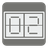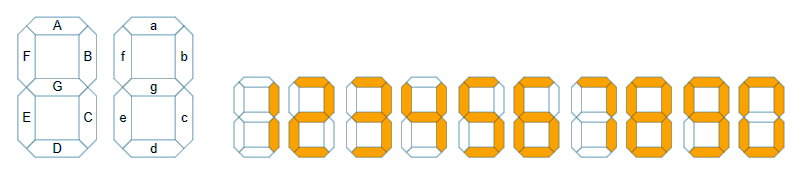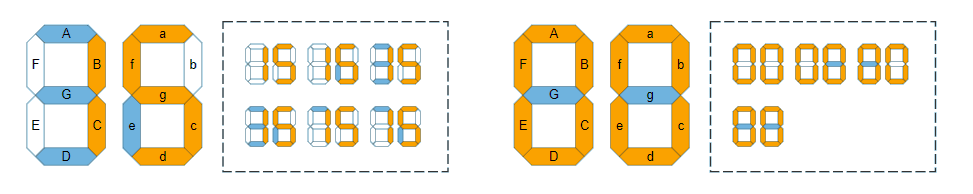Seven Segment
Simple
English JA

You have a device that uses a Seven-segment display to display 2 digit numbers. However, some of the segments aren't working and can't be displayed.

You will be given information on the lit and broken segments. You won't know whether the broken segment is lit or not. You have to count and return the total number that the device may be displaying.

The input is a set of lit segments (the first argument) and broken segments (the second argument).

• Uppercase letters represent the segments of the first out two digit number.
• Lowercase letters represent the segments of the second out two digit number.
• topmost: 'A(a)', top right: 'B(b)', bottom right: 'C(c)', bottommost: 'D(d)', bottom left: 'E(e)', top left: 'F(f)', middle: 'G(g)'Example:

```seven_segment({'B', 'C', 'b', 'c'}, {'A'}) == 2    #11, 71
seven_segment({'B', 'C', 'a', 'c', 'd', 'f', 'g'}, {'A', 'D', 'G', 'e'}) == 6    #15, 16, 35, 36, 75, 76
seven_segment({'A', 'B', 'C', 'D', 'E', 'F', 'a', 'b', 'c', 'd', 'e', 'f'}, {'G', 'g'}) == 4    #0, 8, 80, 88```Input: Two arguments. The first one contains the lit segments as a set of letters representing segments. The second one contains the broken segments as a set of letters representing segments.

Output: The total number that the device may be displaying.

Precondition:

• all(re.match('[A-Ga-g]', s) for s in lit | broken)
• len(lit & broken) == 0